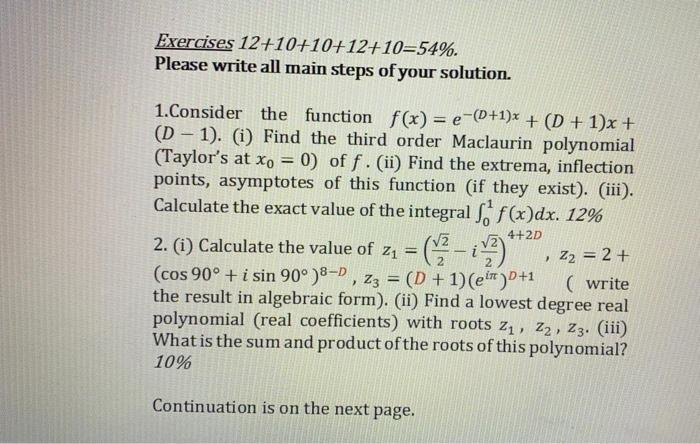### Create an Account

Home / Questions / Exercises 12+10+10+12+10=54%. Please write all main steps of your solution. 1. Consider th...

# Exercises 12+10+10+12+10=54%. Please write all main steps of your solution. 1. Consider the function f(x) = e-(D+1)x + (D+1)x + (D – 1). (i) Find the third order Maclaurin polynomial (Taylor's at Xo =

Exercises 12+10+10+12+10=54%. Please write all main steps of your solution. 1. Consider the function f(x) = e-(D+1)x + (D+1)x + (D – 1). (i) Find the third order Maclaurin polynomial (Taylor's at Xo = 0) of f. (ii) Find the extrema, inflection points, asymptotes of this function (if they exist). (iii). Calculate the exact value of the integral f(x)dx. 12% 2. (i) Calculate the value of zı (cos 90° + i sin 90°) 8-D , Zz = (D + 1) (Ft) +1 ( write the result in algebraic form). (ii) Find a lowest degree real polynomial (real coefficients) with roots Z1, Z2, Z3. (iii) What is the sum and product of the roots of this polynomial? 10% 4+2D , 22 = 2 + Continuation is on the next page.
D=2Apr 28 2021 View more View LessSubscribe To Get Solution